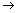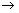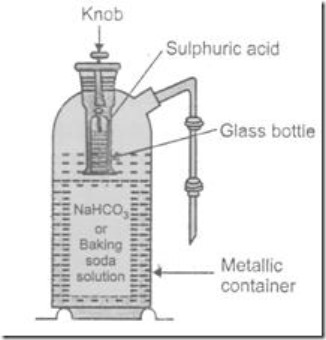# Chemistry X | Acid, Bases and Salts: NCERT Solved Questions

## Acid, Bases and Salts: NCERT Solved Questions

### Question 1. You have been provided with three test tubes. One of them contains distilled water and the other two contain an acidic solution and a basic solution respectively. If you are given only red litmus paper, how will you identify the contents of each test tube ?

We will dip the red litmus paper in all the test tubes, the test tube in which red litmus changes to blue., contains, basic solution. Now dip the so changed blue litmus paper in other two test tubes, the test tube in which blue litmus changes to red is acidic solution and the third test tube will contain distilled water.

### Question 2. Why should curd and sour substances not be kept in brass and copper vessels ?

Curds and other sour substances contain acids which react with brass and copper to form toxic substances which are not suitable for health and consumption. So, it is advisable not to store curds and other sour substances in brass or copper vessels.

### Question 3. Which gas is usually liberated when an acid reacts with a metal ? Illustrate with an example. How will you test the presence of the gas ?

Usually hydrogen gas is liberated when an acid reacts with a metal. For example, when zinc granules react with dilute sulphuric acid, zinc, sulphate and hydrogen are produced.

Zn (s) + H2S04 (aq)ZnS04 (aq) + H2 (g)

Hydrogen gas burns with a ‘pop’ sound.

### Question 4. A metal compound A reacts with dilute hydrochloric acid to produce effervescence. The gas evolved extinguishes a burning candle. Write a balanced chemical equation for the reaction if one of the compounds formed is calcium chloride.

Metal compound A reacts with dilute hydrochloric acid to form a gas which extinguishes burning candle means produced gas is carbon dioxide. Other product is calcium chloride, so compound A is carbonate or hydrogen carbonate of calcium. We can present the reaction as follows:

### Question 5. Why do HCl, HNO3 etc. show acidic character in aqueous solutions while solutions of compounds like C2H5OH and glucose do not show acidic character ?

HCl, HNO3 show acidic character in aqueous solutions because they produce H+ (aq) ions, but solutions of C2H5OH and glucose do not produce H+ ions, so they do not show acidic character.

### Question 6. Why does an aqueous solution of an acid conduct electricity ?

An aqueous solution of an acid conduct electricity because in aqueous solution acid produces cations and anions.

### Question 7. Why does dry HCl gas not change the colour of dry litmus paper ?

H+ions are responsible for acidic character of HC1 and change of litmus paper. H+ ions are formed in the presence of water only. That’s why, dry HCl gas cannot change the colour of dry litmus paper.

### Question 8. While diluting the acid why is it recommended that acid should be added to water and not water to the acid ?

Mixing water and acid is an exothermic reaction. When water is added to acid due to small amount of water, evolved heat will change it to vapour and acid will spill out and may cause injury. So, it is advised to mix acid to water, not water to acid.

### Question 9. How is the concentration of hydronium ions (H3O+) affected when a solution of an acid is diluted ?

when a solution of an acid is diluted, the concentration of hydronium ions (H30+) is decreased.

### Question 10. How is concentration of hydroxide ions (OH–) affect when excess base is dissolved in a solution of sodium hydroxide ?

When excess base is dissolved in a solution of sodium hydroxide concentration of hydroxide ions (OH) is increased.

### Question 11. You have two solutions A and B. pH of solution A is 6 and pH of solution B is 8. Which solution has more hydrogen ion concentration ? Which of the above solution is acidic and which one is basic ?

Solution having more hydrogen ion concentration – Solution A.

Acidic solution – Solution A.

Basic solution – Solution B.

### Question 12. What effect does the concentration of H+ (aq) have on the acidic nature of the solution ?

Acidic nature of a solution is directly proportional to the H+ (aq) ion concentration. If H+ (aq) ion concentration of one solution is higher than the other, it will be more acidic than the other.

### Question 13. Do basic solution also have H+ (aq) ions ? If yes, then why are they basic ?

Yes, basic solution also have H+ (aq) ions. If a solution has H+ (aq) ions and (OH) ions concentration is neutral and when (OH) ions > H+ ions, solution is basic.

### Question 14. Under what soil conditions do you think a farmer would spread or treat the soil of his fields with quick lime (calcium oxide) or slaked lime (calcium hydroxide) or chalk (calcium carbonate).

Quick lime, slaked lime and chalk are basic in nature, so soil should be treated using these substances when the soil is acidic in nature

### Question 15. What is the common name of compound CaOCl2 ?

The common name of compound CaOCl2 is bleaching powder.

Dry slaked lime.

### Question 17. Name the sodium compound which is used for softening of hard water.

Washing soda (sodium carbonate) is used for softening of hard water.

### Question 18. What will happen if the solution of sodium hydrogen carbonate is heated ? Give the equation of the reaction involved.

On heating NaHCO3 changes to Na2CO3

(a) 1

(b) 4

(c) 5

(d) 10

(a) NaCl

(b) HCl

(c) LiCl

(d) KCl.

(a) 4 mL

(b) 8 mL

(c) 12 mL

(d) 16 mL.

(a) Antibiotic

(b) Analgesic

(c) Antacid

(d) Antiseptic.

### (d) Dilute hydrochloric acid reacts with iron filings

.

(a) Zinc (s) + Sulphuric acid (aq)Zinc sulphate (aq) + Hydrogen (g)

Zn (s) + H2S04 (aq)ZnS04 (aq) + H2 (g)

(b) Magnesium (s) + Hydrochloric acid (aq) —> Magnesium chloride (aq) + Hydrogen (g)

Mg (s) + 2HC1 (aq)MgCl2 (aq) + H2 (g)

(c) Aluminium (s) + Sulphuric acid (aq)Aluminium sulphate (aq) + Hydrogen (g)

2A1 (s) + 3H2S04 (aq)A12(S04)3 (aq) + 3H2 (g)

(d) Iron (s) + Hydrochloric acid (aq)Iron chloride (aq) + Hydrogen (g)

2Fe (s) + 6HC1 (aq)2FeCl3 (aq) + 3H2 (g)

### Question 25. With the help of a chemical reaction explain how a soda-acid fire extinguisher helps in putting out a fire.

In soda-acid fire extinguisher, which contains a solution of sodium hydrogen carbonate surrounding a glass bottle containing sulphuric acid.

In case of fire, the knob (provided at the top of extinguisher) is pressed, thereby bottle breaks and the two solutions come in contact, thereby liberating carbon dioxide gas.

2 NaHCO3 + H2SO4Na2SO4 + 2 H2O + 2 CO2

The liberated carbon dioxide forces a stream of effervescing liquid on the fire. In this way, carbon dioxide surrounds the combustible substances and cut­off the supply of air, thereby it assists to put out the fire.### Question 27. Why does distilled water not conduct electricity whereas rain water does.

Rain water dissolves atmospheric carbon dioxide to form carbonic acid (H2C03) which forms H+ions and due to presence of H+ ions, rain water conducts electricity, but a distilled water cannot.

### Question 28. Why do acids do not show acidic behaviour in the absence of water?

Acids show acidic behaviour when they form H+ (aq) ions which are not formed in the absence of water.

### (f) arrange the pH in increasing order of hydrogen ion concentration.

Solution Showing pH

(a) Neutral 7

(b) Strongly alkaline: 11

(c) Strongly acidic: 1

(d) Weakly acidic: 4

(e) Weakly alkaline: 9

(f) pH in increasing order of hydrogen ion concentration: 11 <9<7<4< 1.

### Question 30. Equal lengths of magnesium ribbons are taken in test tubes A and B. Hydrochloric acid (HCl) is added to tube A while acetic acid (CH3COOH) is added to test tube B. In which test tube will the fizzing occur more vigorously and why ?

The fizzing will occur more vigorously in test tube A because HCl is a strong acid and it produces more number of H+ ions.

### Question 31. Fresh milk has a pH of 6. How do you think the pH will change as it turns into curd ? Explain your Answer

When milk changes to curd its pH will decrease due to the formation lactic acid.

### (b) Why does the milk take a long time to set as curd.

(a) Addition of baking soda (alkaline in nature) makes milk more alkaline, i.e., pH of milk increase about

(b) The pH required for fermentation of milk is about 5-6 and we know that on addition of baking sour milk makes it alkaline {i.e., pH>7). Hence, milk takes a long time to set as curd.

### Question 33. Plaster of Paris should be stored in moisture-proof container. Explain why ?

Plaster of Paris changes to a hard substance reacting with water. That’s why, it is stored in a moisture- proof container.

### Question 34. What is a neutralization reaction ? Give two examples.

Neutraliztion is the interaction of an acid and base with the formation of salt
Example :

HCl (aq) + NaOH (aq)NaCl (aq) + H20 (I)

H2S04 (aq) + Ca (OH)2 (aq)CaS04 (aq) + 2H20 (I)

### Question 33. Give two important uses of washing soda and baking soda.

Uses of washing soda :

(i) It is used in the manufacture of soap, glass and paper.

(ii) It is used in the production of sodium compound.

Uses of baking soda :

(i) It is an important ingredient of baking powder.

(ii) It is also used as antacid.

# Chemistry X | Periodic Classification of Elements | Trends in the Modern Periodic Table

Trends in the Modern Periodic Table

Valency : As you know, the valency of an element is determined by the number of valence electrons present in the termost shell of its atom.

Activity 5.6

1. How do you calculate the valency of an element from its electronic configuration?
2. What is the valency of magnesium with atomic number 12 and sulphur with atomic number 16?
3. Similarly find out the valencies of the first twenty elements.
4. How does the valency vary in a period on going from left to right?
5. How does the valency vary in going down a group?

Atomic size: The term atomic size refers to the radius of an atom. The atomic size may be visualised as the distance between the centre of the nucleus and the outermost shell of an isolated atom. The atomic radius of hydrogen atom is 37 pm (picometre, 1 pm = 10–12m). Let us study the variation of atomic size in a group and in a period.

Activity 5.7

1. Atomic radii of the elements of the second period are given below:

Period II elements : B Be O N Li C

Atomic radius (pm) : 88 111 66 74 152 77

1. Arrange them in decreasing order of their atomic radii.
2. Are the elements now arranged in the pattern of a period in the Periodic Table?
3. Which elements have the largest and the smallest atoms?
4. How does the atomic radius change as you go from left to right in a period?

You will see that the atomic radius decreases in moving from left to right along a period. This is due to an increase in nuclear charge which tends to pull the electrons closer to the nucleus and reduces the size of the atom.